Chapter 2: Linear Equations

# 2.1 Elementary Linear Equations

Solving linear equations is an important and fundamental skill in algebra. In algebra, there are often problems in which the answer is known, but the variable part of the problem is missing. To find this missing variable, it is necessary to follow a series of steps that result in the variable equalling some solution.

# Addition and Subtraction Problems

To solve equations, the general rule is to do the opposite of the order of operations. Consider the following.

Example 2.1.1

Solve for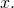1.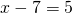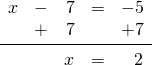2.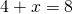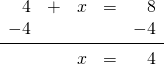3.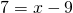4.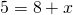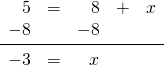# Multiplication Problems

In a multiplication problem, get rid of the coefficient in front of the variable by dividing both sides of the equation by that number. Consider the following examples.

Example 2.1.2

Solve for1.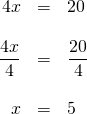2.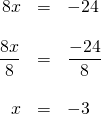3.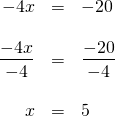# Division Problems

In division problems, remove the denominator by multiplying both sides by it. Consider the following examples.

Example 2.1.3

Solve for1.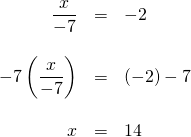2.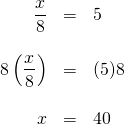3.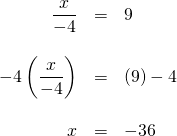# Questions

For questions 1 to 28, solve each linear equation.

1.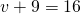2.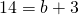3.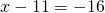4.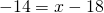5.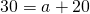6.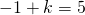7.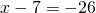8.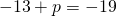9.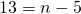10.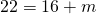11.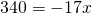12.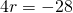13.14.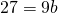15.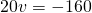16.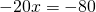17.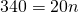18.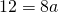19.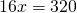20.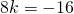21.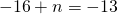22.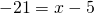23.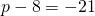24.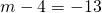25.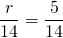26.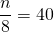27.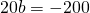28.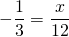# Extra Reading and Instructional Videos